### Total 38 Videos found in Category "AP Calculus I"

Title
 1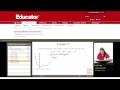Calculus: Absolute Minima and Maxima 2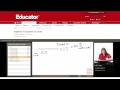Calculus: Algebraic Evaluation of Limits 3Calculus: Applications of Rates of Change 4Calculus: Applied Optimization 5Calculus: Area Between Curves 6Calculus: Average and Instantaneous Rates of Change 7Calculus: Average Value of a Function 8Calculus: Chain Rule 9Calculus: Compositions of Functions 10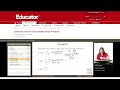Calculus: Continuity 11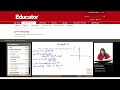Calculus: Curve Sketching 12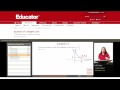Calculus: Equation of a Tangent Line 13Calculus: First Derivative Test, Second Derivative Test 14Calculus: Formal Definition of a Limit 15Calculus: Graphing Derivatives 16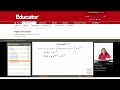Calculus: Higher Derivatives (Find nth Derivative) 17Calculus: Hyperbolic Trigonometric Function Derivatives 18Calculus: Implicit Differentiation 19Calculus: Intermediate Value Theorem 20Calculus: Inverse Trigonometric Functions 21Calculus: L Hopital Rule 22Calculus: Limit Definition of the Derivative 23Calculus: Limit Investigations (Two Sided Limit) 24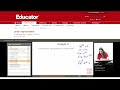Calculus: Linear Approximation 25Calculus: Logarithmic and Exponential Function Derivatives 26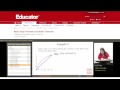Calculus: Mean Value Theorem 27Calculus: Newton Method 28Calculus: Power Rule 29Calculus: Product Rule 30Calculus: Quotient Rule 31Calculus: Related Rates (Ladder Sliding Down a Wall) 32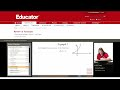Calculus: Review of Functions (Properties of e) 33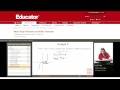Calculus: Rolle Theorem 34Calculus: Separable Differential Equations 35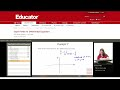Calculus: Slope Fields for Differential Equations 36Calculus: Trigonometric Derivatives 37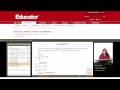Calculus: Volume Disks and Washers 38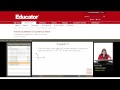Calculus: Volume with Cylindrical Shells

Say and share some thing about these videos...